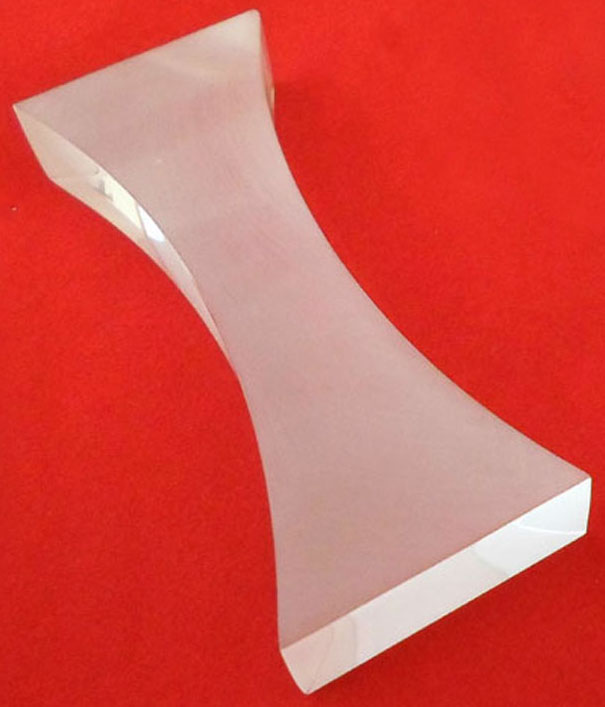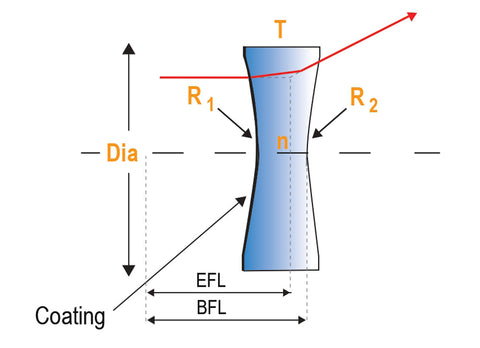Swati Talwar

HS-PS4-1

double concave lens importance

double concave lens uses

biconvex and biconcave

# Double Concave Lenses Study Guide

A bi-concave (double concave) lens has the same radius of curvature on both sides of the lens and functions like a plano-concave lens by diverging collimated incident light.

Table of content

# INTRODUCTION

Definition of Double Concave Lens

Double-Concave Lenses (DCV or Biconcave Lenses) are employed in beam expansion, image reduction, and light projection applications. These lenses are also great for extending an optical system's focal length. Optical lenses with negative focal lengths are double-concave lenses, which feature two concave surfaces.

The concave lens deflects a straight ray from the light source and reduces an upright virtual image. It has the ability to create both real and virtual images. At least the inner surface of the concave lens is curved.

Concave lenses are also called diffuse lenses because the center is curved inward, and the edges are curved outward to diffuse the light. They are used to treat myopia because they make distant objects look smaller.Source

## SOME IMPORTANT POINTS ABOUT DOUBLE CONCAVE LENSSource

• A divergent lens.
• All surfaces of the lens have a curved shape.
• The center is thinner than the edges.
• Negative focal length is a term used to describe the length of a lens.
• There are two main points.
• Creates a virtual representation that is smaller than the object.

## USES OF DOUBLE CONCAVE LENS

If the object-image conjugate ratio is close to 1: 1 and the input beam is converging, a biconcave lens is best. The exit ray of the virtual image diverges (on the object side). The distance between the virtual image and the lens is called the focal length.

These are commonly used in existing systems such as beam expanders and projection systems to increase illumination and focal length. Suitable when the object and image are about 1: 1 conjugate to the conjugate input beam.

## DIFFERENCE BETWEEN BICONVEX AND BICONCAVE LENS

Biconvex Lens:

• Converging
• Both sides of the lens have a curved shape.
• It is thicker in the center than at the edges.
• focus length that is positive
• There are two focal points.
• Creating a physical or virtual image
• Lenses that can be used as magnifying or condensing lenses
• Used as magnifiers or objectives
• Telescopes, monoculars, microscopes, binoculars, cameras, and projectors are examples of imaging systems.
• Eyes of humans
• Image exchangers use it.

Biconcave Lens:

• Diverging
• Both sides of the lens have a curved shape.
• It's thinner in the middle than it is at the edges.
• The focal length that is negative
• There are two focal points.
• Creating a virtual representation.
• Telescopes, peepholes, light projection, and binoculars are all used to enlarge objects.
• Used in Presbyopia.

# SUMMARY

• A lens is a transparent substance with at least one curved surface (glass or plastic). The word "lens" comes from the Latin word "lentil," which refers to a bean with the shape of a Bi-convex lens.
• As light rays pass through the lens, they bend in a specific way, either converging to a given point or diverging as if from a specific point, depending on the lens shape.

## FAQs

Q. What is another name for a double concave lens?

Concave-concave lens or Biconcave lens.

Q. What is a double lens?

If your prescription glasses have two distinct sections in the lenses, you most certainly have what is known as bifocals. This is referred to as a double lens.

Q. In which category is a double concave lens placed?

This type of lens is a diverging lens.

We hope you enjoyed studying this lesson and learned something cool about Double Concave Lens! Join our Discord community to get any questions you may have answered and to engage with other students just like you! We promise, it makes studying much more fun!😎

## REFERENCE

1. Optical Lens: https://3doptix.com/blogs/education/optical-lens-types-and-main-differences Accessed 19th April 2022.
2. Bi-concave Lens: https://www.newport.com/c/bi-concave-lenses#:~:text=Bi%2DConcave%20(Double%2DConcave,collimated%20incident%20light%20to%20diverge. Accessed 19th April 2022.